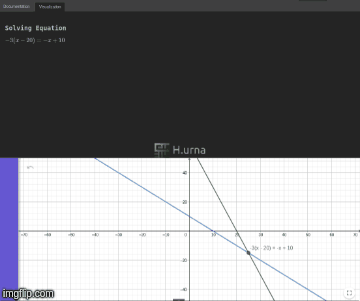# Linear equation solver and graphic visualizer with step-by-step explanations

• Many people think that equations and algebra are obscure and use some esoteric languages. Truth is that equations are actually relatively simple concepts; knowing the base rules of the game and a bit of practice, you can learn to manipulate and solve them in a snap.

Hurna propose you here a mathematical equation solver and visualizer with step-by-step explanation. It can help in learning concepts. It can help giving you graphical visualization of algebraic operation. It can be used as a simple day-to-day calculator or for double-checking the solution. In short, it makes learning and teaching math very easy.Indeed, it solves your math equations step-by-step like a teacher would.

Whether you are a teacher, a parent or a student, you may found this tool very useful.

Jump in Hurna Explorer to practice and visualize yourself any equations.
https://demo.hurna.io/#path=equation_solver/linear